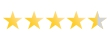# DATA SCIENCE USING R PROGRAMMING 6 WEEKS TRAINING IN NOIDA4.5 out of 5 based on 1699 Votes.

This is a complete tutorial to learn data science and machine learning using R. By the end of this tutorial, you will have a good exposure to building predictive models using machine learning on your own.R is a free programming language and software environment for statistical computing and graphics. The R language is widely used among statisticians and data miners for developing statistical software and data analysis. R is an implementation of the S programming language combined with lexical scoping semantics inspired by Scheme.S was created by John Chambers while at Bell Labs. You can learn this technology from beginning to end in Ducat.To get quality training, you need to learn from trainers highly skilled in this technology. Professionals handling projects in real time will assist students and fresher's to understand challenges and working scenario in the industry. Ducat is the Best Data Science Using R Programming institute in noida.

• Descriptive statistics
• Inferential Statistics
• Statistical data analysis
• Variables
• Sample and Population Distributions
• Interquartile range
• Central Tendency
• Normal Distribution
• Skewness.
• Boxplot
• Five Number Summary
• Standard deviation
• Standard Error
• Hypothesis

## Objectives:

This module introduces you to some of the important keywords in R like Business Intelligence, Business
• Analytics, Data and Information. You can also learn how R can play an important role in solving complex analytical problems.
• This module tells you what is R and how it is used by the giants like Google, Facebook, etc.
• Also, you will learn use of 'R' in the industry, this module also helps you compare R with other software
• in analytics, install R and its packages.

## Topics

• Understanding Business Analytics and R
• Compare R with other software in analytics
• Install R
• Perform basic operations in R using command line

## R objects and Data types

• Vector
• Matrix
• Array
• Factor
• List
• Data Frame
• Factors
• NA/NAN
• Explicit Coercion
• Lists
• Missing Value

## Inbuilt Functions of R

• Environment functions
• Statistical Functions
• li>Text Functions
• Mathematical Functions
• Writing Data into R
• Files connection

• If
• For
• Repeat
• While
• Next
• Return

• Lappy
• Tappy
• Split
• Mappy
• Apply

## Date and time in R

• Dates in R
• Time in R
• Operation on Dates and Time on R

## Basic Graphs in R

• Creating a graph
• Density Plot
• Dot Plot
• Bar Plot
• Line Charts
• Pie Charts
• Box Plot
• Scatter Plot
• Histogram
• Normal QQ Plot

• Graphical Parameters
• Lattice Graphs
• Combining Plot
• Ggplots Graph
• Probability Graphs
• Correlograms

## PREDICTIVE MODELLING IN MARKETING

• Personal loans cross selling
• Life insurance
• Regression
• Variable selection
• Multiple regression
• Logistic regression
• Multi-colinearity

## BUSINESS ENVIRONMENT with DATA SCIENCE

• To implement the statistical solutions that the team comes up with, sound programming and insight generation.
• Communicate the insights using business-friendly presentations
• Actionable insights
• CORRELATION
• Histogram, scatter plot, boxplot